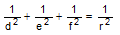Problem 213. Triangle, Incircle, Inradius, Semicircles, Common Tangents In the figure below, the circle O of radius r is tangent to the sides of triangle ABC at D, E, and F. If d, e, and f are the common tangents to the semicircles of diameter AD, DB, BE, EC, CF, and FA, prove that:.  View or post a solution.Home | Geometry | Problems | 211-220 | Email | View or post a solution